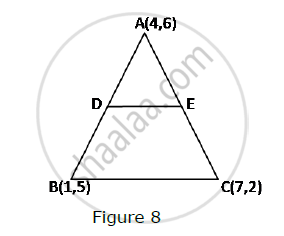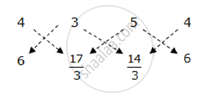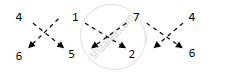# In Fig. 8, the vertices of ΔABC are A(4, 6), B(1, 5) and C(7, 2). A line-segment DE is drawn to intersect the sides AB and AC at D and E respectively such that (AD)/(AB)=(AE)/(AC)=1/3 Calculate th area of ADE and compare it with area of ΔABCe. - Mathematics

In Fig. 8, the vertices of ΔABC are A(4, 6), B(1, 5) and C(7, 2). A line-segment DE is drawn to intersect the sides AB and AC at D and E respectively such that (AD)/(AB)=(AE)/(AC)=1/3 Calculate th area of ADE and compare it with area of ΔABCe.#### Solution

(AD)/(AB)=(AE)/(AC)=1/3

:.(AB)/(AD)=(AC)/(AE)=3

:.(AD+DB)/(AD)=(AE+EC)/(AE)=3

:.1+(DB)/(AD)=1+(EC)/(AE)=3

:.(DB)/(AD)=(EC)/(AE)=2

:.(AD)/(DB)=(AE)/(EC)=1/2

∴ AD:DB = AE :EC = 1: 2

So, D and E divide AB and AC respectively in the ratio 1:2.

So the coordinates of D and E are

((1+8)/(1+2),(5+12)/(1+2))=(3,17/3)=1/2|(4xx17/3+3xx14/3+5xx6)-(3xx6+5xx17/3+4xx14/3)|

=1/2|(68/3+14+30)-(18+85/3+56/3)|

=1/2|((68+42+90)/3)-((54+85+56)/3)|

=1/2|(200/3)-(195/3)|

=1/2xx5/3

=5/6Area of ΔABC

=1/2|(4xx5+1xx2+7xx6)-(1xx6+7xx5+4xx2)|

=1/2|(20+2+42)-(6+35+8)|

=1/2|64-49|

=15/2

:.(

Concept: Area of a Triangle
Is there an error in this question or solution?
2015-2016 (March) All India Set 1

Share